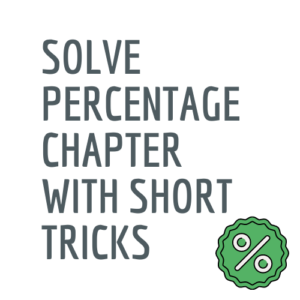Percentage questions for SSC cgl

It is the most crucial chapter in maths. If you understand percentage basic or concept, you can easily manage your time in the exam. Here, we discuss various percentage problems and answer with quick math shortcut tricks methods below in this article. We request all students to read carefully. First, you can attempt your percentage question and answer for ssc cgl with the basic concept and note how much time it will take to finish. Now goes through this article of percentage problems tricks explained in shortcut method. You will see improvement in your time after attempting with the help of these percentage tricks.

IMPORTANT POINT

If you are preparing for any competitive exam, then the maths section is the most important. If you work hard and practice well, you will surely get maximum marks than any other section in the exam. So, the first step is to brush up on your primary concept of every topic of math.

Solve previous year’s question paper with time management. You have to solve maximum questions in less time. Practice and practice are the only way of it. Here in this article, we are providing some percentage tricks for the math percentage chapter. There are six types of examples listed below. You can go through it and do some practice. After practice well, you will see that your timing to solve these percentage questions with tricks improves.

Basically, in a previous article, we provide the profit and loss and work and time tricks

now, we give a percentage chapter of math that is very useful in all exams, and 8-10 no. of questions are asked from that chapter.

With the help of percentage tricks, we can apply it to various forms like ratio, profit loss, time and distance, graphs, pie charts, etc., these are are the form of a percentage, and if you clear the basic concept, it will be beneficial in many ways.

So, first, what is a percentage.first what is percentage

DEFINITION
a percentage is a number or ratio expressed as a fraction of 100.

if we have to convert percentage into fraction than it is divide by 100.

Example 1:- if we write 45% then its equal to 45/100 or in fraction 9/20 or in decimal 0.45

if we have to convert fraction into percentage we have to multiple with 100.
Example 2 :- if we write 3/5 in fraction it is equal to 60%
3/5×100=60.

READ AND LEARN FOLLOWING TABLE as also known as conversion table of percentage. Learn this table it help you a lot to solve many problem.

the following fraction in red colour are MOST IMPORTANT.

 Fraction Percentage 1/1 100% 1/2 50% 1/3 33.33% 1/4 25% 1/5 20% 1/6 16.66% 1/7 14.28% 1/8 12.5% 1/9 11.11% 1/10 10% 1/15 6.66% 1/20 5% 1/25 4% 1/30 3.33% 1/40 2.5% 1/50 2% 1/60 1.66% 1/75 1.33% 1/80 1.25% 1/90 1.11% 1/100 1%

by this conversion table we can change fraction into percentage and percentage into fractionEX:- if we have to find 2/5 in %?

In the table we know that 1/5=20%
then, if we multiply both by 2
then we have 1/5×2 = 2×20%

so, you only to know basic fraction and percentage that given above.
in the inverse
Ex. If we have to find out 1/15 fraction in percentage%?

In the table we have 1/5 = 20%
then both side multiple by 1/3

we get 1/15 = 6.66%

SOME BASIC PERCENTAGE PROBLEMS TRICKS AND SHORTCUTS THAT IS ALWAYS ASKED IN THE EXAMS

PROBLEM ON PERCENTAGE

TYPE 1.
if there is increase of X% and subsequently X% decrease then there is always loss / decrease in the condition.
ex. If rohan salary is increase by 50% and subsequently decrease by 50%. How much percentage loss?

ans. By trick —-  50×50/100= 25% decrease/loss

TYPE 2.
if A is P% more than B. then B is less than by A
with P/(100+P)x100

ex. If radha earning is 25% more than sita. Then sita earning is how many percentage less then by radha?
ans. By trick —- 25/(100+25)x100=20%
TYPE 3.
If A is P% less than B. then B is more than by A
with P/(100-P)x100

ex. If golu age is 20% less than gita than gita age is how many percentage more than golu?
ans. By trick —- 20/(100-20)x100=25%
TYPE 4.
if there is p% increase in price . how much % decrease his consumption so that his expenditure on it does not change.
formula P/(100+P)x100

ex. If petrol price increase by 25%. How much percentage a person reduced his consumption so that his expenditure on it does not increase?
ans. 25/(100+25)x100=20%

TYPE 5.

if there is p% decrease in price . how much % increase his consumption so that his expenditure on it does not change.
formula P/(100-P)x100

ex. If there is 30% decrease in eggs price. How much a person increased his consumption so that his expenditure on it does not increase?
ans. 30/(100-30)x100=42.85

TYPE 6.
Ex. If suman need 36% minimum mark to passed a exam but she score 24% mark and fail by 9 marks. What is the total mark?
ans. Difference in % = difference in mark
In that 36%-24%=12%
that is 12%=9marks
so, total mark = 9/12×100= 75

these are the some examples that are usually asked in the chapter of percentage in ssc exams.